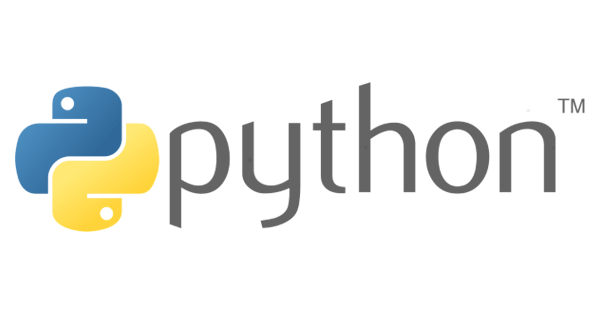# Python if...elif...else 條件判斷語法Python 與其它程式一樣有「條件判斷語法」，但 Python 的 if 較不同的地方在於它使用 elif 而不是 else if，而且也沒有 switch 語法。

## if 組合方式

if 判斷式可以使用以下幾種方式組合。

if 單一條件判斷：

``````if 條件式:
# 語句...``````

if…else 單一條件判斷：

``````if 條件式:
# 語句...
# 上述條件不成立執行
else:
# 語句...``````

if…elif 多重條件判斷：

``````if 條件式 1:
# 語句...
elif 條件式 2:
# 語句...``````

if…elif…else 多重條件判斷：

``````if 條件式 1:
# 語句...
elif 條件式 2:
# 語句...
elif 條件式 3:
# 語句...
.
.
# 上述條件式都不成立執行
else:
# 語句...``````

## 範例程式

``````if True:
print('if True')
'''
if True
'''

if False:
print('if True')
else:
print('else True')
'''
else True
'''

if False:
print('if True')
elif True:
print('elif True')
'''
elif True
'''

if False:
print('if True')
elif True:
print('elif True')
else:
print('else True')
'''
elif True
'''``````

``````score = int(input('請輸入考試分數 (1-100)：'))

if score > 100:
print('請勿亂輸入！')
elif score >= 90 and score <= 100:
print('成績：A+')
elif score >= 80 and score < 90:
print('成績：B+')
elif score >= 70 and score < 80:
print('成績：B')
else:
print('成績：C')
'''

'''``````